Courses

NCERT Solutions - Work and Energy, Science, Class 9 Notes | Study Class 9 Science by VP Classes - Class 9

Class 9: NCERT Solutions - Work and Energy, Science, Class 9 Notes | Study Class 9 Science by VP Classes - Class 9

The document NCERT Solutions - Work and Energy, Science, Class 9 Notes | Study Class 9 Science by VP Classes - Class 9 is a part of the Class 9 Course Class 9 Science by VP Classes.
All you need of Class 9 at this link: Class 9

Q1. A force of 7 N acts on an object. The displacement is, say 8 m, in the direction of the force. Let us take it that the force acts on the object through the displacement. What is the work done in this case?
Ans.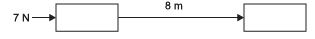F = 7 N
s = 8 m
W = F × s = 7
N × 8 m
W = 56 J
∴ Work done is 56 J.

Q2. When do we say that work is done?
Ans.
Work is said to be done when force applied on an object shows the displacement in that object.
W = F × s.

Q3. Write an expression for the work done when a force is acting on an object in the direction of its displacement.
Ans.
Work done = W
Force = F
Displacement = s
∴ Work done is equal to the product of the force and displacement.
∴ W = F × s

Q4. Define 1 J of work.
Ans.
1 Joule of work is said to be done when force of 1 Newton acts on a body and shows displacement of 1 metre.

Q5. A pair of bullocks exerts a force of 140 N on a plough. The field being ploughed is 15 m long. How much work is done in ploughing the length of the field?
Ans.
Force = 140 N s = 15 m W = ?
∴ W = F × s = 140 N × 15 m = 2100 Nm
W = 2100 J
∴ Work done in ploughing the length of the field is 2100 J.

Q6. What is the kinetic energy of an object?
Ans.
The energy possessed by a body by the virtue of its motion is called kinetic energy. Every moving object possesses kinetic energy. A body uses kinetic energy to do work. Kinetic energy of hammer is used in driving a nail into a log of wood, kinetic energy of air is used to run wind mills, etc.

Q7. Write an expression for the kinetic energy of an object.
Ans.
If a body of mass mis moving with a velocity v, then its kinetic energy Eis given by the expression,

Ek= 1/2 mv2

Its SI unit is Joule (J).

Q8. The kinetic energy of an object of mass, m moving with a velocity of 5 ms– 1 is 25 J. What will be its kinetic energy when its velocity is doubled? What will be its kinetic energy when its velocity is increased three times?
Ans.
K.E. = 25 J, v = 5 m/s
K.E. = (1/2) mv
25 = (1/2) m (5)2
∴ m = 2 kg

(i) If velocity is doubled i.e., v = 10m/s, m = 2kg
K.E. =(1/2) mv2 =(1/2) (2) × (10)2 = 100 J,
K.E will become four times.

(ii) If velocity is 3 times, v = 15 m/s,m = 2 kg
K.E. =(1/2) mv2
= (1/2) × 2 × (15)2
= 225 J, K.E will become nine times.

Q9. What is power?
Ans.
Power is the rate of doing work or the rate of transfer of energy. If W is the amount of work done in time t, then power is given by the expression,

Power= Work/Time = Energy/Time

P = W/T

It is expressed in watt (W).

Q10. Define 1 watt of power.
Ans.
Power is said to be 1 watt when 1 Joule of work is done in 1 second.

Q11. A lamp consumes 1000 J of electrical energy in 10 s. What is its power?
Ans.
P = ?
W = 1000 J

P = W/t = 1000/10

∴ Power is 100 watt.

Q12. Define average power.

Ans. Average power is defined as the total energy consumed divided by the total time taken.

NCERT textual Questions solved

Q1. Look at the activities listed below. Reason out whether or not work is done in the light of your understanding of the term ‘work’.
(a)Suma is swimming in a pond.
(b) A donkey is carrying a load on its back.
(c)A wind-mill is lifting water from a well.
(d)A green plant is carrying out photosynthesis.
(e)An engine is pulling a train.
(f) Food grains are getting dried in the sun.
(g)A sailboat is moving due to wind energy.
Ans.
(a) Suma pushes water in backward direction and the displacement is in forward direction, work is said to be done.
W = −ve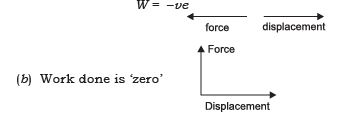(c) Force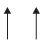Displacement of water W = +ve

(d) No displacement, hence no work is done

(e) Work is done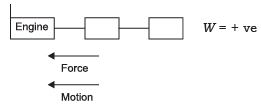(f ) No displacement, hence no work is done.

(g) Work is done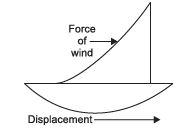Q2. An object thrown at a certain angle to the ground moves in a curved path and falls back to the ground. The initial and the final points of the path of the object lie on the same horizontal line. What is the work done by the force of gravity on the object?
Ans.
Work done by the force of gravity on the object is zero. Force of gravity acts in the vertical downward direction and the distance covered by the object is in the horizontal direction. As there is no displacement in the direction of force, hence the work done is zero.

Q3. A battery lights a bulb. Describe the energy changes involved in the process.
Ans.
Chemical energy of battery → transformed into heat energy of bulb filament, which is further transformed into light energy.

Q4. Certain force acting on a 20 kg mass changes its velocity from 5 m/s to 2 m/s.
Calculate the work done by the force.
Ans.
mass = 20 kg u = 5 m/s v = 2 m/s W = ?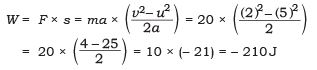Q5. A mass of 10 kg is at a point A on a table. It is moved to a point B. If the line joining A and B is horizontal. What is the work done on the object by the gravitational force? Explain your answer.
Ans.
Force acts on a body of mass 10 kg to move it horizontally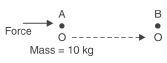from A to B as shown above. Work is said to be done on the object by the force.
But the work done on the object by the gravitational force is zero.
Since work done by gravitational force depends upon change in vertical height.

Q6. The potential energy of a freely falling object decreases progressively. Does this violate the law of conservation of energy? Why?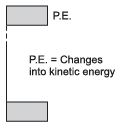Ans. A body at a particular height has maximum potential energy, when it falls the potential energy is transformed into kinetic energy, when the object is about to touch the ground, the body has maximum velocity and hence the kinetic energy is maximum.
Energy is not lost but it gets transformed into different forms. It abides by the Law of Conservation of Energy.

Q7. What are the various energy transformations that occur when you are riding a bicycle?
Ans.
Muscular energy gets transformed into mechanical energy.

Q8. Does the transfer of energy take place when you push a huge rock with all your might and fail to move it? Where is the energy you spend going?
Ans.
When rock is pushed with all might the energy does not allow the rock to move. No work is said to be done. The energy gets transformed in the form of heat energy.

Q9. A certain household has consumed 250 units of energy during a month. How much energy is this in joules?
Ans.
Commercial unit of energy = 250 units
1 unit = 1 kWh
∴ 250 units = 250 kWh
1 kWh = 3.6 × 106 J
∴ 250 units = 250 × 3.6 × 106 J
Energy in Joules = 9 × 10J

Q10. An object of mass 40 kg is raised to a height of 5 m above the ground. What is its potential energy? If the object is allowed to fall, find its kinetic energy when it is half-way down.
Ans.
Mass = 40 kg

h = 5 m
P.E. = ?
P.E. = mgh = 40 × 10 × 5 Q
g = 10 m/s2
P.E. = 2000 J
When the object falls the potential energy gets transformed into kinetic energy.
When the object is half way down
∴P.E. will become half i.e., 20200 = 1000 J and P.E. = K.E.
Kinetic Energy = 1000J

Q11. What is the work done by the force of gravity on a satellite moving around the earth? Justify your answer.
Ans.
As the force acting on the body is perpendicular to the direction of displacement,
∴ Work done is zero.

Q12. Can there be displacement of an object in the absence of any force acting on it? Think. Discuss this question with your friends and teacher.
Ans.
If an object is in a state of motion, then as per Newton’s first law of motion in the absence of any external force acting on it, the object will maintain its motion along a given straight line. So the object will have displacement. However, if the object is initially at rest then there can be no displacement, the work done by person is zero.

Q13. A person holds a bundle of hay over his head for 30 minutes and gets tired. Has he done some work or not? Justify your answer.
Ans.
Work is said to be done when force is applied on a body and the body shows the displacement.
In this case force is applied on hay bundle but there is no displacement of it.
Hence no work is said to be done on the hay.

Q14. An electric heater is rated 1500 W. How much energy does it use in 10 hours.
Ans.
Power = 1500 W → 1.5 kW
time = 10 hour
Energy = ?
Energy = Power × Time taken = 1.5 × 10
= 15 kWh
∴ Energy required = 15 kWh

Q15. Illustrate the law of conservation of energy by discussing the energy changes which occur when we draw a pendulum bob to one side and allow it to oscillate. Why does the bob eventually come to rest? What happens to its energy eventually? Is it a violation of the law of conservation of energy?
Ans.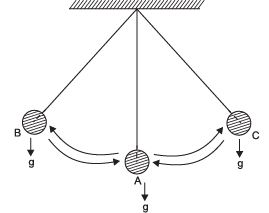Let ‘A’ be the mean position of pendulum. When it is raised to point ‘B’ and released,

it starts oscillating from B to A, A to C and back to A. It repeats this motion again and again.
At point B and C, height of pendulum is maximum, hence the potential energy at these points is maximum.
During oscillation, when pendulum moves from B − A − C, its maximum speed is at A, hence the maximum kinetic energy is at A, and potential energy at this point is minimum.
This shows the potential energy at B is transformed to kinetic energy at A and again this energy is transformed into potential energy at C.
This transformation of energy goes on and the pendulum oscillates, it does not violate the law of conservation of energy.
Bob comes to rest because there are three forces acting on it,
(i) Gravitational force exerted by earth ‘g’ which tries to bring the bob at rest.
(ii) Air resistance force that acts opposite the motion of pendulum.
(iii) Restoring force, the pendulum is tied at a point, the upward tension pull also tries to get the pendulum to its original position.

Q16. An object of mass, m is moving with a constant velocity, v. How much work should be done on the object in order to bring the object to rest?
Ans.
The mass of the object = m
Velocity = v
K.E. = (1/2) mv2 – This is the energy of the object with which the body is moving, hence same amount of energy is required to stop the body.
∴ Work done on the object in order to bring the object to rest = K.E. =(1/2) mv2

Q17. Calculate the work done required to be done to stop a car of 1500 kg moving at a velocity of 60 km/h.
Ans.
mass = 1500 kg

v = 60 km/h =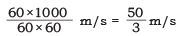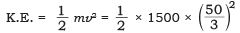Q18. In each of the following a force, F is acting on an object of mass m. The direction of displacement is from west to east shown by the longer arrow. Observe the diagrams carefully and state whether the work done by the force is negative, positive or zero.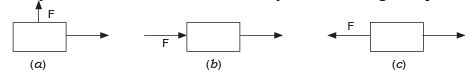Ans. (a) Incase ( a ) , the force F is perpendicular to the displacement , hence work done = 0. (b) In case
(b), the force F is in same direction as that of displacement, hence work done is positive. (c) In case
(c), the force F is opposite to the direction of displacement, hence work done is negative.

Q19. Soni says that the acceleration in an object could be zero even when several forces are acting on it. Do you agree with her? Why?
Ans
. Yes, when several forces are acting on a body, if the forces are opposite to each other, the acceleration produced can be zero.

Q20. Find the energy in kwh consumed in 10 hours by four devices of power 500 W each.
Ans.
Power = 500 W = 0.5 kW
time = 10 hours
Devices = 4
∴ Energy consumed in kWh = 0.5 kW × 10 hours × 4 = 20 kWh

Q21. A freely falling object eventually stops on reaching the ground. What happens to its kinetic energy?
Ans.
When a body is freely falling the energy remains conserved. Potential energy is transformed into kinetic energy. But when the body reaches the ground, its velocity, (v = 0) becomes zero. Hence the kinetic energy is also zero.

The document NCERT Solutions - Work and Energy, Science, Class 9 Notes | Study Class 9 Science by VP Classes - Class 9 is a part of the Class 9 Course Class 9 Science by VP Classes.
All you need of Class 9 at this link: Class 9Use Code STAYHOME200 and get INR 200 additional OFF Use Coupon Code

Top Courses for Class 9Class 9 Science by VP Classes

75 docs|14 tests

Top Courses for Class 9Track your progress, build streaks, highlight & save important lessons and more!

,

,

,

,

,

,

,

,

,

,

,

,

,

,

,

,

,

,

,

,

,

,

,

,

,

,

,

;